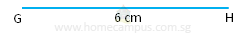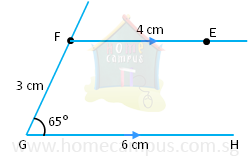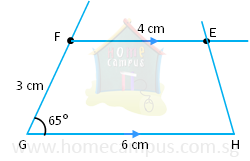## How to Draw a Trapezium?

Practice Unlimited Questions

#### 1. Draw a trapezium EFGH so that FE // GH, ∠FGH = 65°, GH = 6 cm, FG = 3 cm and FE = 4 cm.

Step 1: Draw a straight line GH 6 cm long.Step 2: Using a protractor draw an angle of 65° from Point G. Mark the Point F so that FG = 3 cm.Step 3: Using a ruler and a set square draw a line FE so that it is parallel to GH. Mark the Point E so that FE = 4 cm.Step 4: Join Point E to Point HG.EFGH is the required trapezium.